Year 9 Interactive Maths - Second Edition

A quadrilateral is a closed plane figure bounded by four line segments.  E.g. The figure ABCD shown here is a quadrilateral.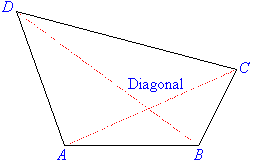A line segment drawn from one vertex of a quadrilateral to the opposite vertex is called a diagonal of the quadrilateral.  For example, AC is a diagonal of quadrilateral ABCD.

There are six basic types of quadrilaterals:

###### 1.  Rectangle

Opposite sides of a rectangle are parallel and equal.  All angles are 90º.

###### 2.  Square

Opposite sides of a square are parallel and all sides are equal.  All angles are 90º.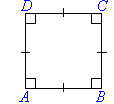###### 3.  Parallelogram

Opposite sides of a parallelogram are parallel and equal.  Opposite angles are equal.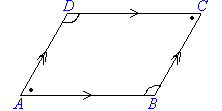###### 4.  Rhombus

All sides of a rhombus are equal and opposite sides are parallel.  Opposite angles of a rhombus are equal.  The diagonals of a rhombus bisect each other at right angles.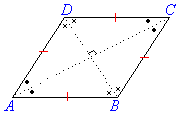###### 5.  Trapezium

A trapezium has one pair of opposite sides parallel.  A regular trapezium has non-parallel sides equal and its base angles are equal, as shown in the following diagram.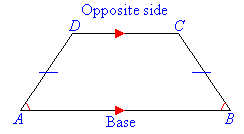###### 6.  Kite

Two pairs of adjacent sides of a kite are equal, and one pair of opposite angles are equal.   Diagonals intersect at right angles.  One diagonal is bisected by the other.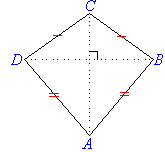#### Theorem 3

Prove that the angle sum of a quadrilateral is equal to 360º.

##### Proof: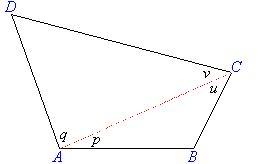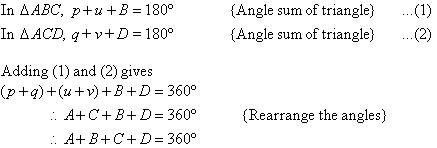Hence the angle sum of a quadrilateral is 360º.

### Applying Properties of Angles in Quadrilaterals

The theorems we have proved can be used to prove other theorems.  They can also be used to find the values of the pronumerals in a problem.

#### Example 13

Find the value of the pronumeral x in the following diagram.  Give reasons for your answer.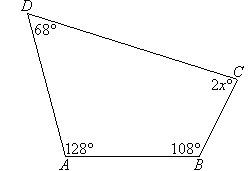##### Solution: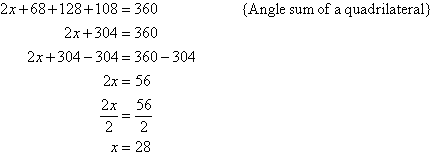#### Example 14

Find the value of the pronumeral x in the following diagram.  Give reasons for your answer.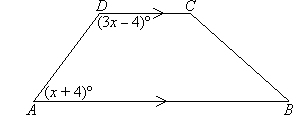##### Solution: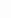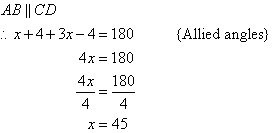#### Example 15

Find the value of each of the pronumerals in the following diagram.  Justify your answers.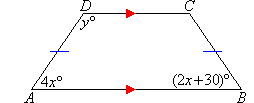##### Solution:

AD = BC, so the trapezium is regular.

Therefore, the base angles are equal.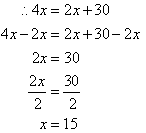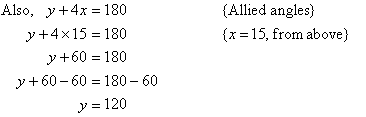#### Example 16

Find the value of each of the pronumerals in the following diagram.  Justify your answers.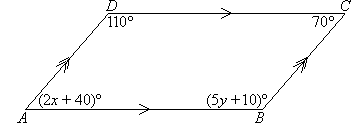##### Solution: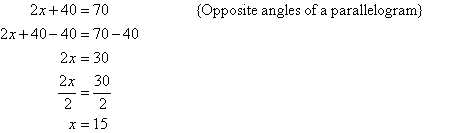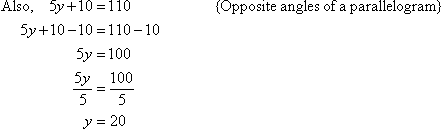So x = 15, y = 20.

#### Example 17

Find the value of each of the pronumerals in the following diagram.  Justify your answers.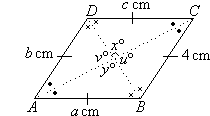##### Solution: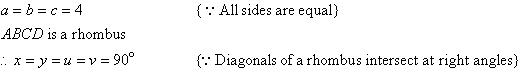#### Example 18

Find the value of each of the pronumerals in the following diagram.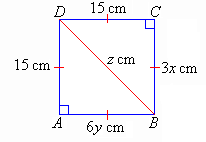##### Solution: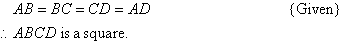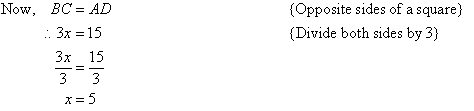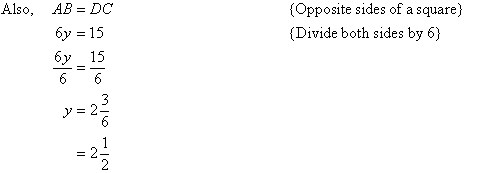By Pythagoras' theorem from triangle ABD, we have: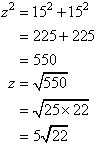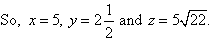#### Example 19

Find the value of the pronumeral x in the following diagram.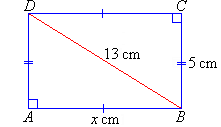##### Solution: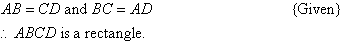By Pythagoras' theorem from triangle ABD, we have: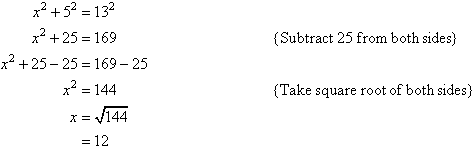So, x = 12.